## Integrals

Integral expression can be added using the \int_{lower}^{upper} command.

Note, that integral expression may seems a little different in inline and display math mode.

LaTeX code Output
Integral $$\int_{a}^{b} x^2 \,dx$$ inside text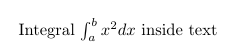$\int_{a}^{b} x^2 \,dx$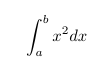## Multiple integrals

To obtain double/triple/multiple integrals and cyclic integrals you must use amsmath and esint (for cyclic integrals) packages.

LaTeX code Output
\begin{gather*}
\iint_V \mu(u,v) \,du\,dv
\\
\iiint_V \mu(u,v,w) \,du\,dv\,dw
\\
\iiiint_V \mu(t,u,v,w) \,dt\,du\,dv\,dw
\\
\idotsint_V \mu(u_1,\dots,u_k) \,du_1 \dots du_k
\end{gather*}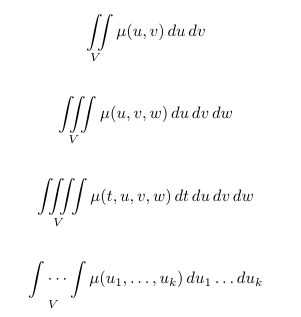$\oint_V f(s) \,ds$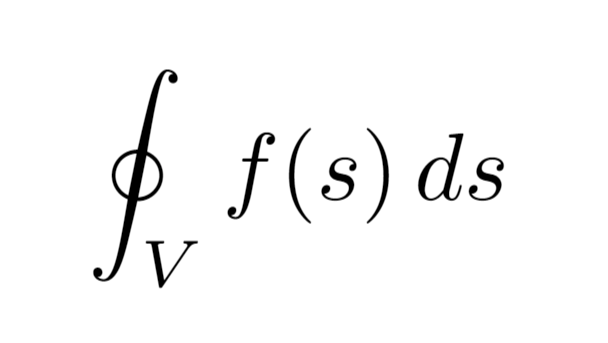## Sums and products

Like integral, sum expression can be added using the \sum_{lower}^{upper} command.

LaTeX code Output
Sum $\sum_{n=1}^{\infty} 2^{-n} = 1$ inside text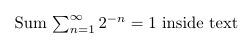$\sum_{n=1}^{\infty} 2^{-n} = 1$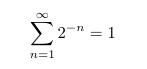In similar way you can obtain expression with product of a sequence of factors using the \prod_{lower}^{upper} command.

LaTeX code Output
Product $\prod_{i=a}^{b} f(i)$ inside text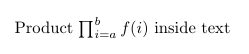$\prod_{i=a}^{b} f(i)$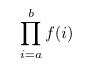## Limits

Limit expression can be added using the \lim_{lower} command.

LaTeX code Output
Limit $\lim_{x\to\infty} f(x)$ inside text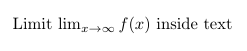$\lim_{x\to\infty} f(x)$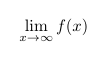## Examples

Here is an example LaTeX document:

\documentclass{article}
\title{Integrals, Sums and Limits}
\author{Overleaf}
\date{}
\usepackage{amsmath}

\begin{document}

\maketitle

\section{Integrals}

Integral $$\int_{a}^{b} x^2 dx$$ inside text.

\medskip

The same integral on display:
$\int_{a}^{b} x^2 \,dx$
and multiple integrals:
\begin{gather*}
\iint_V \mu(u,v) \,du\,dv
\\
\iiint_V \mu(u,v,w) \,du\,dv\,dw
\\
\iiiint_V \mu(t,u,v,w) \,dt\,du\,dv\,dw
\\
\idotsint_V \mu(u_1,\dots,u_k) \,du_1 \dots du_k
\\
\oint_V f(s) \,ds
\end{gather*}

\section{Sums and products}

Sum $$\sum_{n=1}^{\infty} 2^{-n} = 1$$ inside text.

The same sum on display:
$\sum_{n=1}^{\infty} 2^{-n} = 1$

Product $$\prod_{i=a}^{b} f(i)$$ inside text.

The same product on display:
$\prod_{i=a}^{b} f(i)$

\section{Limits}

Limit $$\lim_{x\to\infty} f(x)$$ inside text.

The same limit on display:
$\lim_{x\to\infty} f(x)$

\end{document}